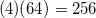# High School Math : Understanding Fractional Exponents

## Example Questions

### Example Question #2 : Fractional Exponents

Convert the exponent to radical notation.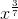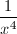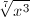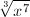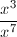Explanation:

Remember that exponents in the denominator refer to the root of the term, while exponents in the numerator can be treated normally.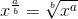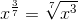### Example Question #1 : Understanding Fractional Exponents

Which of the following is equivalent to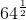?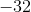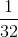Explanation:

By definition, a number raised to thepower is the same as the square root of that number.

Since the square root of 64 is 8, 8 is our solution.

### Example Question #1 : Fractional Exponents

Simplify the expression: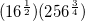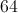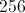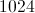Explanation:

Remember that fraction exponents are the same as radicals.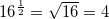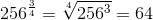A shortcut would be to express the terms as exponents and look for opportunities to cancel.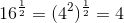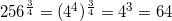Either method, we then need to multiply to two terms.